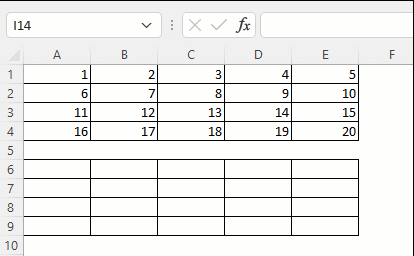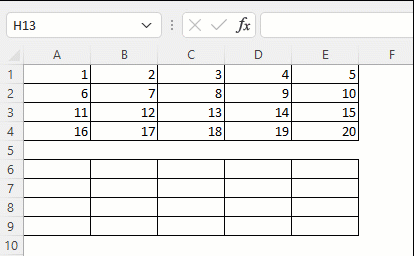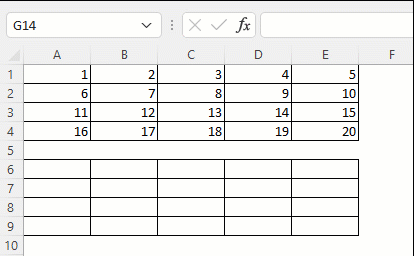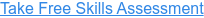Back to Blog# Absolute, Relative and Mixed References

Published
Mar 12, 2021 7:06:00 PM

Formulae in Excel contain references to other cells or ranges of cells. There are three types of references that can be used: absolute, relative or mixed.

#### What are absolute, relative and mixed references?

When we create a formula in Excel we typically reference other cells to bring the values from those cells into our formula.

The referencing of the cell can be relative, absolute or mixed. The type of cell reference is only important when you copy (or drag) the formula from one cell to another.

#### How do I create absolute, relative and mixed references in Excel?

Absolute and mixed referencing is applied by putting a \$ symbol before the letter (column) or the number (row) in the cell reference.

The F4 function key is a shortcut for cycling through the references:

1. Absolute reference
2. Row reference
3. Column reference
4. Relative reference

#### Let's look at each of them in turn:

Relative cell reference

Both the row and column references are free to change when the formula is copied to another cell.

Example: =A1Note that there is not a \$ symbol in front of the letter (column) or the number (row).

Absolute cell reference

Both the row and column references are fixed and do not change when the formula is copied to another cell.

Example: =\$A\$1Note that the \$ symbol is in front of both the letter (column) and number (row).

Mixed - Row cell reference

The row reference is fixed and does not change when the formula is copied to another cell.

Example of fixed row: =A\$1Note that the \$ symbol is in front of both the letter (column) and number (row).

Mixed - Column cell reference

The column reference is fixed and does not change when the formula is copied to another cell.

Example of fixed column: =\$A1Note that the \$ symbol is in front of the letter (column).

Read more about the absolute and relative references in Excel on the Microsoft support page here.

#### Take our free 30-question skills assessment

See where you stand with your Excel, financial modelling, data visualisation & analytics, and modelling tech skills